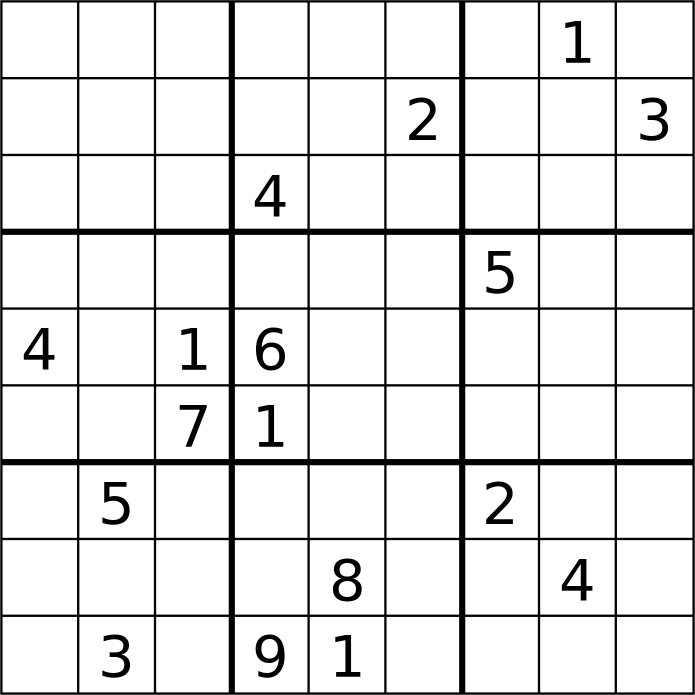# The hardest problem in computer science (Part 1)

The P vs. NP problem is perhaps the biggest unsolved question in computer science – but an answer would have profound implications for mathematics, cryptography, cancer research, nurse roster scheduling, and sudoku. [1 of 2]LithiumFlash [CC BY-SA]Every now and then I like to tackle a topic that’s a little more complex than “hur hur Porky Pig swore.” This time, it’s the question of whether P and NP are the same or not. Prove this question one way or the other and we will have an answer to some of the most intractable problems in the world. Also some mathematicians will give you a million dollars.

Because it’s such a big topic, I’m going to split the blog post into two parts. Today, I’m going to try to explain P and NP, and to do that we’ll begin with the idea of the algorithm.

An algorithm is essentially a set of instructions for solving a problem. For example, say you want to alphabetise your library by title. You would take all of the book titles and enter them into a computer running a sorting algorithm. It would go through a series of internal steps to rearrange them, and then it would give you back the full alphabetised list.

(Incidentally, there are a lot of sorting algorithms, each of which uses different steps to get to the same result: merge sorts, bubble sorts, bucket sorts, and many more. But that’s a topic for another time.)

At the heart of the P vs. NP problem are two questions: How long does it take an algorithm to find the result? How long does it take to check the result once it has been found?

How long will it take to sort your books? Algorithms work step by step, so you can predict how long it will take to solve a particular problem based on the size of the input. To put it another way, the larger your library, the longer it will take to sort it… but not that much longer. As the number of books increases, the time to sort it increases at a fairly steady rate (in mathematical terms, it increases in polynomial time). In computer science, problems of this type are called “P.”

Checking whether the algorithm has sorted your books correctly also takes time. The time it would take to check the answer also increases as the library gets larger, but that too increases at a steady and modest rate. Problems that can be checked easily are called “NP” (for “nondeterministic polynomial time”).

With me so far? Quick recap: problems that can be solved easily = P. Problems that can be checked for accuracy easily = NP. (“Easily” here is defined according to some specific mathematical criteria that I’m skipping over!)

Let’s switch to a different, but related, problem: sudoku puzzles. It is really easy and fast to check a result in sudoku – if there are no repeated numbers in every row and column the solution is correct. But actually solving the sudoku takes a lot more time. I mean, have you tried solving a sudoku puzzle? Fiendish. Computer algorithms also take quite a few steps to figure it out.

This is where we get to the crux of the matter: we’re going to make the sudoku grid larger. Instead of a 9×9 grid, we’ll use a 16×16 grid. The time it takes to check it doesn’t increase by that much. The time it would take to solve it with an algorithm also increases. But it doesn’t increase in that modest fashion we’ve seen before: the time it takes increases exponentially. It’s a computing blow-out! At least, it seems to be the case according to every algorithm we have designed so far.

What this means is that sudoku puzzles are NP (solution is easily checked) but not P (solution is easily found). Or so we think. Most computer scientists and mathematicians believe that many problems like this exist: easy to check but not easy to solve.

There’s just one problem with this belief: they cannot prove it.